# IRR Function

Returns the Internal Rate of Return for a given cash flow

## What is the IRR Function?

The IRR function is categorized under Excel Financial functions. IRR will return the Internal Rate of Return for a given cash flow, that is, the initial investment value and a series of net income values.

The function is very helpful in financial modeling, as it helps calculate the rate of return an investment would earn based on series of cash flows. It is frequently used by businesses to compare and decide between capital projects. One example is when a company is presented with two opportunities: one is investing in a new factory and the second is expanding its existing factory. Using the IRR function, we can estimate the IRRs for both scenarios and check which one is higher than the weighted average cost of capital (WACC) of the business (also known as a hurdle rate ). If both are greater than the cost of capital, we will choose the one that shows a higher IRR and/or Net Present Value (NPV) than the other.

Note: for increased precision, CFI recommends you use the XIRR function

### IRR Formula

=IRR(values,[guess])

The IRR function uses the following arguments:

1. Values (required argument) – This is an array of values that represent the series of cash flows. Cash flows include investment and net income values. Values can be a reference to a range of cells containing values.
2. [Guess] (optional argument) – This is a number guessed by the user that is close to the expected internal rate of return (as there can be two solutions for the internal rate of return). If omitted, the function will take a default value of 0.1 (=10%).

#### Notes

1. The argument value should contain at least one positive and one negative value to calculate the internal rate of return.
2. The IRR function uses the order of the values to interpret cash flows. Hence, it is necessary to enter the payments and income values sequentially.
3. If the array or reference argument contains logical values, empty cells or text, those values are ignored.

### How to use IRR Function in Excel?

As a worksheet function, IRR can be entered as part of a formula in a cell of a worksheet. To understand the uses of the function, let us consider a few examples:

#### Example 1

For an initial investment of 1,000,000, the cash flows are given below: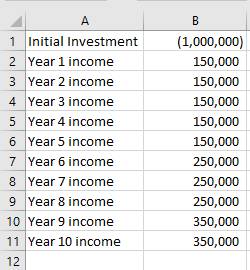The initial investment here is a negative value as it is an outgoing payment. The cash inflows are represented by positive values.

The internal rate of return we get is 14%.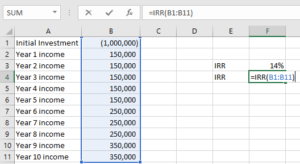#### Example 2

Let’s calculate the CAGR using IRR. Suppose we are given the following information: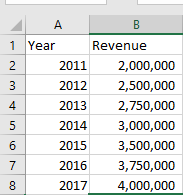The IRR function is not exactly designed for calculating compound growth rate, so we need to reshape the original data in this way: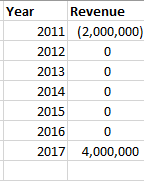The beginning value of the investment should be entered as a negative number. The ending value of the investment is a positive number. All intermediate values are kept as zeros.

Now, we would simply apply the IRR function to calculate the CAGR, as shown below: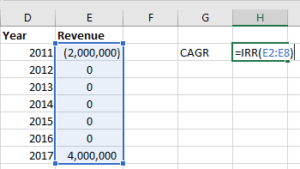The CAGR we get is: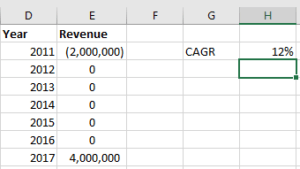Let’s do reverse engineering to check if the resulting value is correct or not.

The formula generally used to calculate CAGR is =((End Value/Start Value)^(1/Periods) -1.

The formula to use will be: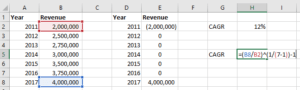The result we get is same as the one we got using IRR function: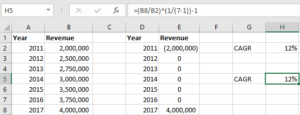### Things to remember about the IRR Function

1. #NUM! error – Occurs when:
1. If the given value array does not contain at least one negative and one positive value
2. The calculation fails to converge after 20 iterations. If the internal rate of return is unable to find a result that works after 20 tries, then the #NUM! error value is returned.
2. IRR is closely related to the NPV (Net Present Value) function. The rate of return calculated by IRR is the discount rate corresponding to a \$0 (zero) NPV.

### Free Excel Course

If you want to learn more about functions and become an expert on them, check out CFI’s Free Excel Crash Course!  Go through our step by step instructions and demonstrations on how to become an Excel power user.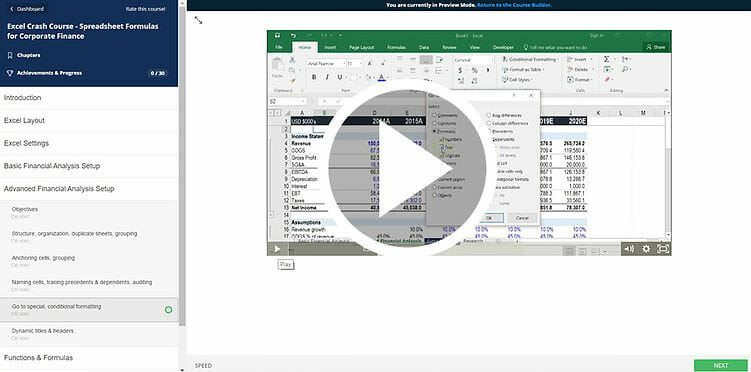Thanks for reading the CFI guide to important Excel formulas! By taking the time to learn and master these functions, you’ll significantly speed up your financial modeling and analysis. To learn more, check out these additional CFI resources:

• XIRR vs IRR
• Modified Internal Rate of Return (MIRR)
• Finacial Modeling Guide
• Financial Analyst Program

### Free Excel Tutorial

To master the art of Excel, check out CFI’s FREE Excel Crash Course, which teaches you how to become an Excel power user.  Learn the most important formulas, functions, and shortcuts to become confident in your financial analysis.

Launch CFI’s Free Excel Course now to take your career to the next level and move up the ladder!